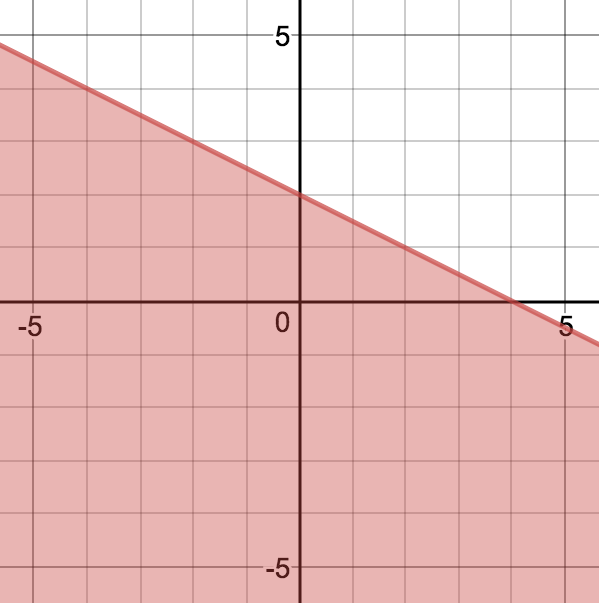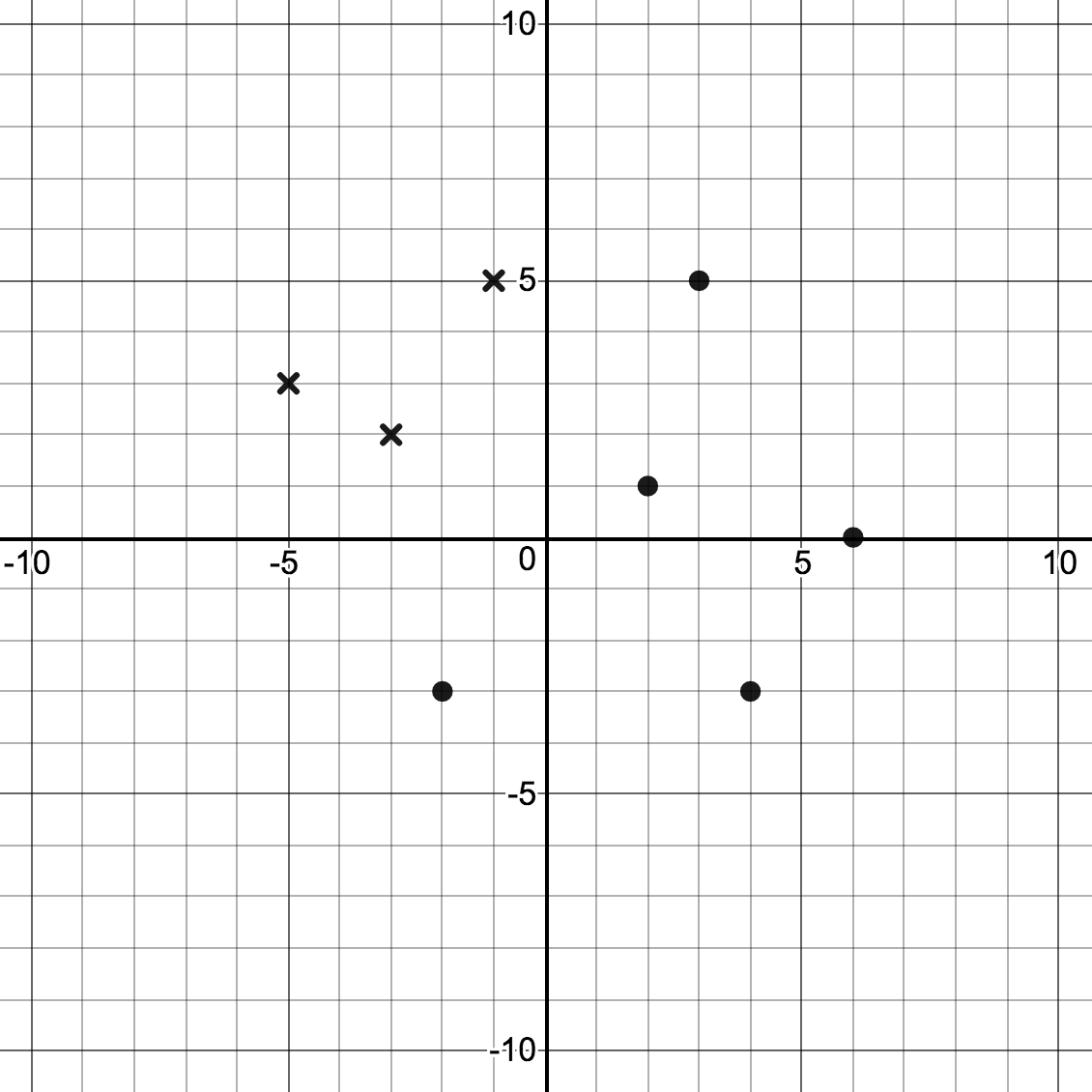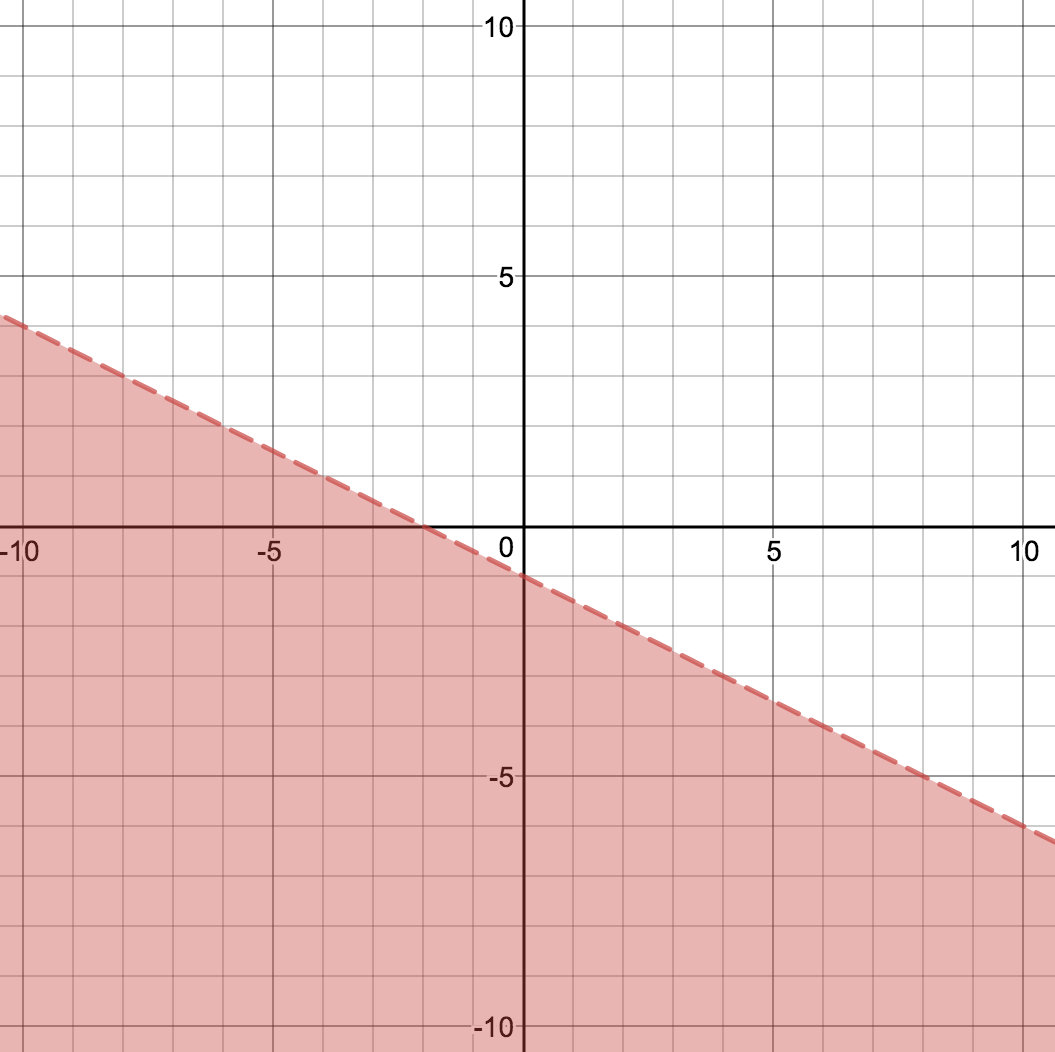# Linear Equations, Inequalities and Systems

## Objective

Write linear inequalities from graphs.

## Common Core Standards

### Core Standards

?

• A.CED.A.3 — Represent constraints by equations or inequalities, and by systems of equations and/or inequalities, and interpret solutions as viable or nonviable options in a modeling context. For example, represent inequalities describing nutritional and cost constraints on combinations of different foods.

• A.REI.D.12 — Graph the solutions to a linear inequality in two variables as a half-plane (excluding the boundary in the case of a strict inequality), and graph the solution set to a system of linear inequalities in two variables as the intersection of the corresponding half-planes.

?

• 7.EE.B.4.B

• 8.EE.B.5

• 8.EE.B.6

## Criteria for Success

?

1. Identify features of linear inequalities from graphs and represent them algebraically.
2. Test points in a linear inequality and verify graphically.
3. Include domain and range restrictions in graphing and writing linear inequalities.

## Anchor Problems

?

### Problem 1

Write the inequality that is graphed below.### Problem 2

Below are several marked points. Write an inequality that does not have the Xs as solutions but has the closed dots as solutions.## Problem Set

?

The following resources include problems and activities aligned to the objective of the lesson that can be used to create your own problem set.